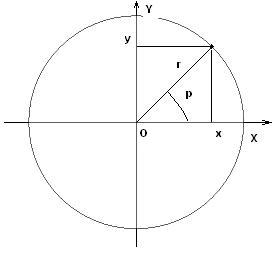# The Polar to Cartesian Coordinate Converter

The Polar to Cartesian Coordinate Converter is an online tool that converts polar coordinates to Cartesian coordinates and vice versa.

Cartesian coordinates, also known as rectangular coordinates, are a two-dimensional coordinate system formed by two perpendicular axes, usually labeled the x-axis and y-axis. The point where the two axes meet is called the origin, which is assigned the coordinates (0,0). Any point in the plane can be identified by an ordered pair of numbers that represent its position relative to the origin. The x-coordinate represents the horizontal position of the point, while the y-coordinate represents the vertical position of the point.

On the other hand, polar coordinates represent a point in the plane by its distance from the origin (r) and the angle it makes with the positive x-axis (θ), measured in a counterclockwise direction. The distance r is called the radial coordinate, and the angle θ is called the angular coordinate or polar angle. The polar angle is usually measured in degrees or radians.Calculators below convert from polar to cartesian coordinates and vice versa. It is assumed that both systems' origin points are the same, and the polar axis is directed along the positive direction of the x-axis. You can find conversion formulas below the calculators.#### Conversion from cartesian coordinates to polar coordinates

Digits after the decimal point: 2

Angular coordinate (azimuth), degrees#### Conversion from polar coordinates to cartesian coordinates

Digits after the decimal point: 2
x-coordinate

y-coordinate

### Polar coordinates to Cartesian coordinates

The conversion between polar coordinates and Cartesian coordinates involves simple trigonometry.
Given a point in polar coordinates (r,θ), we can find its equivalent in Cartesian coordinates (x,y) as follows: and .

Conversely, given a point in Cartesian coordinates (x,y), we can find its equivalent in polar coordinates (r,θ) using the following formulas: and . It is important to note that the angle θ is not unique, as adding or subtracting a multiple of 2π to it results in the same point.

URL copiado para a área de transferência